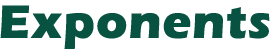The exponential operator, ^, can be made accurate and useful. Here's how.

The exponential operator, ^, performs a very standard mathematical function, although if you are not familiar with mathematics you may not be aware of its potential. Also, there is another byte-saving use that I will save for the end.

The key to making full use of ^ is to realize that in mathematical notation the square root of four is the same as four to the one-half power. In BASIC, you can write either SQR(4) or 4^(1/2). So what good is that? Well, you might want to do a cube root, which would be 8^(1/3). Get the idea? Not believing this works, you might have tried it by now and have noticed that the machine insists that 4^(1/2) is not 2 but rather 1.998... something. It seems strange to accept a wrong answer from a very slow function.

To correct for this inaccuracy, we simply write the instruction INT(4^(1/2)+0.1), and this will return the number 2. In return for this inaccuracy we get the ability to calculate very unusual powers and roots. The above could have been written 4^0.5 and the same answer returned. We could just as easily have 4^0.4321 or 2^2.223 and have gotten an answer correct enough for many calculations. Also, those complex problems such as two to the five-thirds power 2^(5/3) can be calculated with ease. So not only can we do the more common cube roots by using ^(1/3), but we can now also do an entire range of mathematical functions.

It is not only faster but more accurate to write 2*2 rather than 2^2. If we are not doing mathematics, how do we make use of this? How about instead of writing a byte-consuming timing loop for a beep, we simply write A=1^1? If the beep should last longer, then there is always A=1^1^1^1^1^1, etc. It takes quite a while before this simple statement equals the number of bytes consumed by a timing loop. Thus the major drawback to more frequent use of ^ can be turned to our advantage.